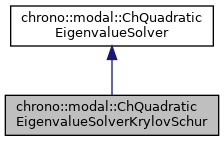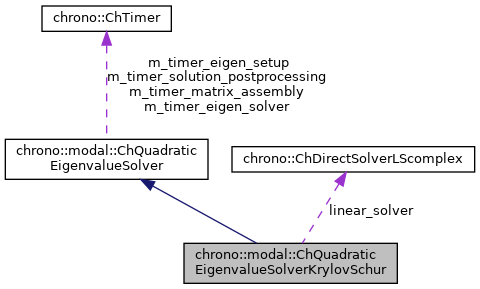## Description

Solves the eigenvalue problem with the Krylov-Schur iterative method.

This is an efficient method to compute only the lower n modes, ex. when there are so many degreees of freedom that it would make a full solution impossible. It uses an iterative method and it exploits the sparsity of the matrices.

#include <ChEigenvalueSolver.h>[legend][legend]

## Public Member Functions

Default: uses Eigen::SparseQR as factorization for the shift&invert, otherwise pass a custom complex sparse solver for faster factorization (ex. More...

virtual bool Solve (const ChSparseMatrix &M, const ChSparseMatrix &R, const ChSparseMatrix &K, const ChSparseMatrix &Cq, ChMatrixDynamic< std::complex< double >> &V, ChVectorDynamic< std::complex< double >> &eig, ChVectorDynamic< double > &freq, ChVectorDynamic< double > &damping_ratio, ChEigenvalueSolverSettings settings=0) const override
Solve the quadratic eigenvalue problem (lambda^2*M + lambda*R + K)*x = 0 s.t. More...

## Public Attributes

ChDirectSolverLScomplexlinear_solver

## Constructor & Destructor Documentation

 chrono::modal::ChQuadraticEigenvalueSolverKrylovSchur::ChQuadraticEigenvalueSolverKrylovSchur ( ChDirectSolverLScomplex * mlinear_solver = 0 )

Default: uses Eigen::SparseQR as factorization for the shift&invert, otherwise pass a custom complex sparse solver for faster factorization (ex.

## ◆ Solve()

 bool chrono::modal::ChQuadraticEigenvalueSolverKrylovSchur::Solve ( const ChSparseMatrix & M, const ChSparseMatrix & R, const ChSparseMatrix & K, const ChSparseMatrix & Cq, ChMatrixDynamic< std::complex< double >> & V, ChVectorDynamic< std::complex< double >> & eig, ChVectorDynamic< double > & freq, ChVectorDynamic< double > & damping_ratio, ChEigenvalueSolverSettings settings = 0 ) const
overridevirtual

Solve the quadratic eigenvalue problem (lambda^2*M + lambda*R + K)*x = 0 s.t.

Cq*x = 0 Returns only the first lower n_modes. If n_modes=0, it return all eigenvalues (performance warning)

< output matrix with eigenvectors as columns, will be resized

< output vector with eigenvalues (real part not zero if some damping), will be resized

< 0 = k-th eigenvalue is real, 1= k-th and k-th+1 are complex conjugate pairs

< 0 = has converged, 1 = hasn't converged

< number of converged eigenvalues

< number of used iterations

< callback for A*v

< initial approx of eigenvector, or random

< size of A

< number of needed eigenvalues

< Krylov restart threshold (largest dimension of krylov subspace)

< max iteration number

< tolerance

Parameters
 M input M matrix R input R matrix K input K matrix Cq input Cq matrix of constraint jacobians V output matrix with eigenvectors as columns, will be resized eig output vector with complex eigenvalues (real part not zero if some damping), will be resized freq output vector with n frequencies [Hz], as f=w/(2*PI), will be resized. damping_ratio output vector with n damping rations r=damping/critical_damping. settings optional: settings for the solver, or n. of desired lower eigenvalues.